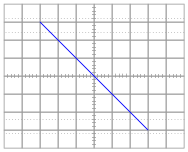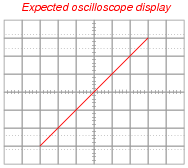# Design Project: Simple Component Curve-Tracer Circuit

## Discrete Semiconductor Devices and Circuits

• #### Question 1

What do each of the oscilloscope axes represent, in real-life terms (variables and units) when used in conjunction with this curve tracer circuit?

• #### Question 2

What mode does the oscilloscope have to be set in, in order to use with this curve tracer circuit?

• #### Question 3

If quantitative accuracy is desired from this curve tracing circuit, the shunt resistor should be precisely 1 kΩ in size. Explain how a precise shunt resistance may be built without the use of precision components (i.e. a special 1 kΩ precision resistor).

• #### Question 4

When testing this circuit for the first time, the student connects a 1 kΩ resistor to the two test leads and gets this result from the oscilloscope display:The student was actually expecting something that looked like this:Upon inspection, nothing appears to be wrong with the wiring of the curve tracer circuit. Explain what the problem is so the student is able to achieve the expected results.